# Derive an Expression for a single-tone FM Wave

## Expression for a single-tone FM Wave

We already know that the FM wave is a sinewave having a constant amplitude and a variable instantaneous frequency.

As the instantaneous frequency is changing continuously, the angular velocity ω of an FM wave is the function of ωc and ωm. Therefore, the FM wave is represented by,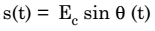…………. (2)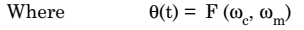……………(3)

As shown in figure 1 , Ec sin θ (t) is a rotating vector.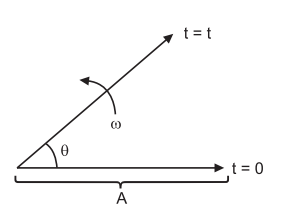Fig.1 : Frequency modulated vector

If Ec is rotating at a constant velocity ‘ω’, then we could have written that θ(t) = ωt.

But, in FM, this velocity is not constant. In fact, it is changing continuously. The angular velocity of FM wave is given as,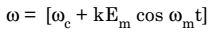Hence, to find ω (t), we must integrate ω with respect to time. Therefore,

As per the definition, Δ f = kE. Thus ,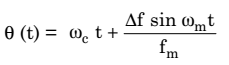Substituting this value of θ (t) in equation (2), we get the equation for the FM wave as under:But,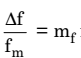is the modulation index of FM wave.

Hence, the equation for FM wave is given as under: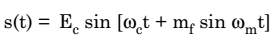This is the expression for a FM wave, where, mf represents the modulation index.